## Introduction to Internal Rate of Return

Companies undertake various projects with the end goal to either increase earnings or cut down costs. These projects often require that the entities make significant investments. In capital budgeting, the management of the business wants to have an estimation of the returns on such investments. The Internal Rate of Return is one method that we can use to rank projects based on their attractiveness for investment. Other factors aside, higher IRR projects are usually preferred, as we expect those to be more profitable for the business.

## What is the Internal Rate of Return

The definition given for IRR is the rate of return at which the present value of the future cash flows is equal to the current value of all costs associated with the investment.

The metric is often used in capital budgeting to value investment opportunities. Typically, we would select the project with the highest Internal Rate of Return.

In financial terms, IRR is the discount rate, at which the Net Present Value of the project is zero. Businesses should look at their IRRs as management sets plans for growth.

## How to Calculate IRR

To calculate the Internal Rate of Return, we can look at the formula for NPV calculation: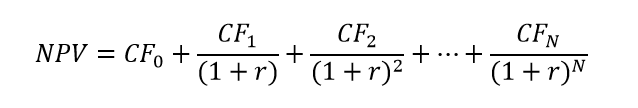Where:

• CF is the cash flow at each period from 0 to N;
• r is the discount rate;
• N is the number of periods.

IRR is the discount rate at which the project has a Net Present Value of zero. NPV of zero means that the total initial and subsequent costs for the project equal the present value of the estimated future cash flows. Adjusting the formula for IRR we get: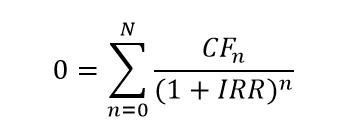Due to the nature of the equation, we can only solve it via trial and error, or by using specialized software solutions, like Excel.

A positive IRR means that the project is profitable, while a negative IRR indicates an undertaking that will be generating a loss.

## Internal Rate of Return in Analysis

Generally, a project with a higher IRR is more attractive. A benefit of the Internal Rate of Return is its uniformity for various types of investments, which makes it an excellent method to rank opportunities. Assuming investment costs are similar, the higher IRR project is most likely to be selected.

IRR is the return rate we expect one investment will generate. Actual return is seldom the same as estimated, but the project with the highest IRR is most likely to be successful.

In reality, we never use IRR solely to make investment decisions. Analysts employ various other methods and techniques and consider an array of quantitative and qualitative factors.

A calculation of Net Present Value usually accompanies the Internal Rate of Return metric, as IRR does not give us actual monetary value. Adding the NPV gives us a better picture of the cost and benefit involved in an investment opportunity.

The method assumes that we can continuously reinvest with the same return rate, which is highly unlikely.

### Modified Internal Rate of Return

IRR is a popular metric, but it tends to overstate profitability and give too optimistic results. Relying on overstated expectations may lead to mistakes in the capital budgeting process.

The Internal Rate of Return gives us an unrealistic picture of how the company reinvests cash flows. On the other hand, the Modified Internal Rate of Return (MIRR) gives us more control over the reinvestment rate assumption for future cash flows.

We can calculate MIRR via the following formula:Where:

• FV – Future value of positive cash flows, with accrued reinvestment rate for each subsequent period;
• PV – Present value of negative cash flows, discounted at the finance rate of the company.

Basic IRR calculation is likely to overstate the future value of cash flows, because of the underlying assumption that reinvestments generate profitability at the IRR. In reality, reinvestments are closer to the cost of capital.

By letting us control the rate at which we reinvest, MIRR mitigates the risk of overstatements.

### IRR and Other Metrics

#### Compound Annual Growth Rate (CAGR)

Financial analysts consider the Internal Rate of Return to be a more robust tool than the CAGR calculation. The Compound Annual Growth Rate measures the rate of return using the beginning and ending values, while IRR considers all cash flows in the investment’s timeline.

However, CAGR has an advantage in that we can easily calculate it by hand.

#### Return on Investment (ROI)

Financial analysts use ROI when evaluating investment opportunities. The ratio looks at the total return of the project, while the calculation of IRR considers annual performance. If we calculate ROI and IRR for a one-year investment, we can expect to get pretty much the same result, but as we extend the timeframe, the two metrics start to deviate more.

The Return on Investment depends on the earnings and costs we will include in the calculation. Therefore it is more susceptible to manipulation. Forecasts of expenses and revenues over more extended periods tend to get highly prone to inaccuracies. It is also hard to quantify the monetary effects of human resources and other factors when estimating the values for the ROI calculation.

## Usage of the Internal Rate of Return

IRR is mostly used to compare expansion projects like growing a current operation, compared against starting new activities. Both options will likely have benefits for the company, but one will probably have a higher positive impact – this will be the logical decision as far as IRR is concerned.

In theory, each opportunity with the Internal Rate of Return above the Weighted Average Cost of Capital of the company is profitable. Firms set Required Rate of Returns (RRR) to benchmark against the return rates of investment opportunities. Management uses this comparison to decide which projects to undertake or forgo. Companies will aim to pursue opportunities with the highest difference between IRR and RRR.

In some cases, investors may compare the Internal Rate of Return to returns on the stock market and decide whether to pursue a project or invest in financial instruments.

## Limitations to the Internal Rate of Return

When applying this technique in our financial analysis, we need to be aware of the following inherent disadvantages:

• We should not trust IRR on its own, as a project might have a lower rate of return, but result in a higher Net Present Value in monetary terms;
• When testing projects of different length, a short-term project might have higher IRR, but result in a low NPV; and a long-term undertaking might have a low IRR, but result in a higher NPV, accumulated over the period;
• People often misuse IRR by assuming the business can reinvest cash flows generated mid-project at the same rate, which is highly unlikely and can lead to overstating the profitability of the investment;
• Large undertakings with volatile cash flows may have several distinct IRR’s and be hard to evaluate.

The above-mentioned Modified Internal Rate of Return takes care of some of these issues by adding the rate at which the company reinvests cash flows to the parameters of the formula. Therefore, MIRR is usually lower than the Internal Rate of Return.

## Example Internal Rate of Return Calculation

To illustrate how we might use the Internal Rate of Return within the premise of financial analysis, let us take a look at the following situation.

We are working in the finance department of a company that has set a hurdle rate of 25%, meaning that management doesn’t consider projects with profitability of less than 25%. The Weighted Average Cost of Capital (WACC) of the company is 10%.

A company is considering two alternative projects where it can invest. Both will cost EUR 400 thousand to implement and will have various maintenance costs, earnings, and durations.

The first project has the following cash flow and period details:The alternative project is shorter and has the following expected future cash flows: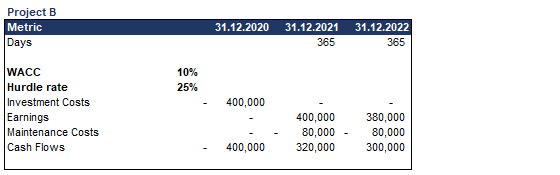As we discussed, we will accompany the Internal Rate of Return calculation with a calculation of NPV.

To get more accurate results, we will employ Excel’s XNPV and XIRR functions.

Here are the metrics calculated for Project A: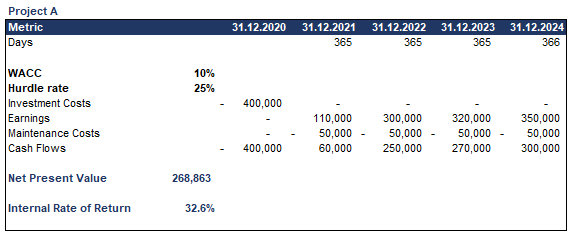And the same parameters for Project B:After we calculated the values for the two alternative projects, let us compare and evaluate which is the more attractive project.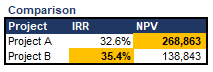We can observe that Project B has a better IRR at 35.4% compared to 32.6% for Project A. However, Project A’s NPV is around two times higher than Project B’s. This is an excellent example of how using only the Internal Rate of Return is not always a good idea, as it provides no monetary context. Calculating the Net Present Value gives us more insight into the two alternatives. In this situation, the company will most likely prefer to go ahead with Project A.

## Conclusion

The Internal Rate of Return can be an excellent basis for investment analysis, as long as we don’t forget about its inherent issues and limitations.

It is also important to remember that IRR only works for projects with an initial cash out-flow (the purchase of the investment).

The metric is best suited to analyze venture capital and private equity investments, which comprise of several cash outflows and one cash inflow at the exit date, generated through an IPO or sale of the investment.

## And Get a FREE Benchmark Analysis Template## FCCA, FMVA

Hi! I am a finance professional with 10+ years of experience in audit, controlling, reporting, financial analysis and modeling. I am excited to delve deep into specifics of various industries, where I can identify the best solutions for clients I work with.

In my spare time, I am into skiing, hiking and running. I am also active on Instagram and YouTube, where I try different ways to express my creative side.